# 决策树原理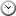2020-03-21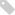AI机器学习, 归类算法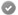浏览次数：1573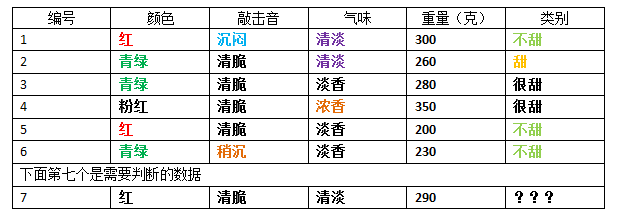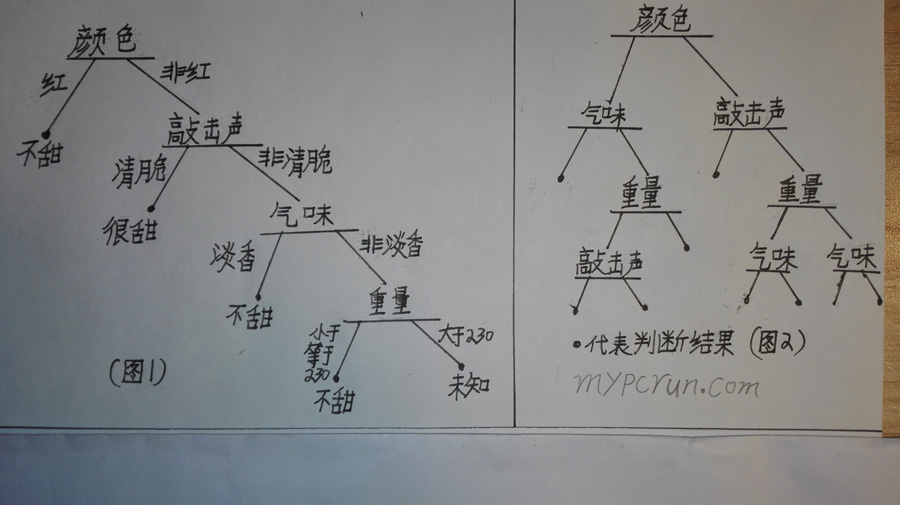“哦，原来这就是决策树，乱来一通也行，只要是个树结构就行，懂了，再见~~”

“道友请留步！你没听我说完，我是说过这个结构可以，但得到的判断结果，会有很大的误差。”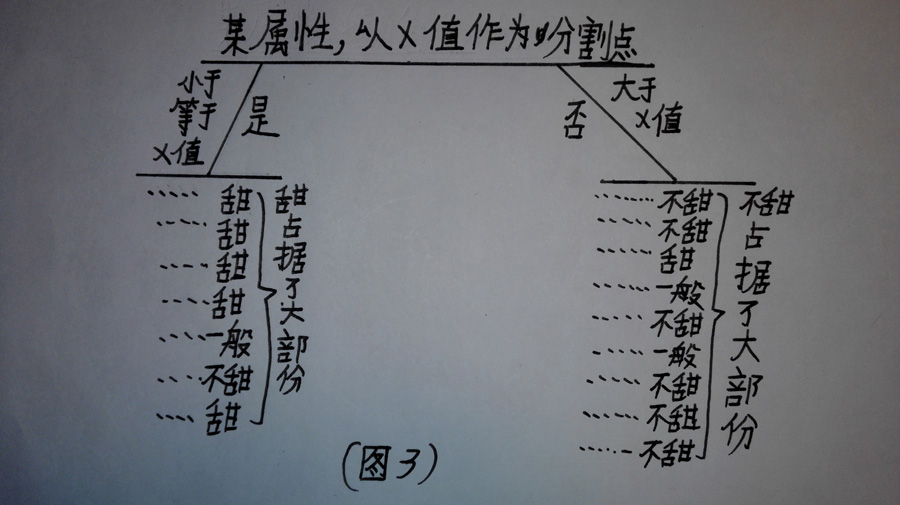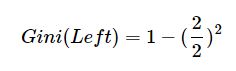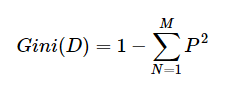N是某个类别，M是类别数量

P(甜|右数据集)=1/4
P(很甜|右数据集)=2/4
P(不甜|右数据集)=1/4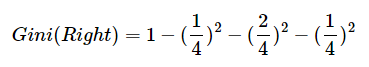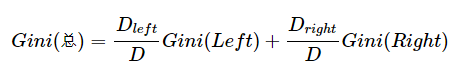D(left)=左边的数据量
D(right)=右边的数据量
D=D(left)+D(right)，其实就是总的数据量

D(left) / D和D(right) / D的用意是，将它们所占的数据比例数作为权重

Gini(颜色|青绿)=0.556
Gini(颜色|粉红)=0.467

Gini(敲击声|沉闷)=0.533
Gini(敲击声|清脆)=0.417
Gini(敲击声|稍沉)=0.533

Gini(气味|清淡)=0.500
Gini(气味|浓香)=0.467
Gini(气味|淡香)=0.556

Gini(重量|?)，哦等等，貌似遇到了问题，重量可是连续值，不是离散值（实际上我也不喜欢这么个称呼，我认为将离散值称为枚举值，还更贴切），怎么办？

P(小于等于300|左边)=1 – (3/5)*(3/5) – (1/5)*(1/5) – (1/5)*(1/5)=14/25

P(大于300|左边)=1 – (1/1)*(1/1)=0

Gini(重量|300)=(5/6) * (14/25) + (1/6) * 0=0.467

Gini(重量|260)=0.444
Gini(重量|280)=0.583
Gini(重量|200)=0.533
Gini(重量|230)=0.417
Gini(重量|350)，这个不用算，因为这个值是最高的，如果还分的话，右子集的数据都是空的了，如果用某一个值划分，当分出的右数据集是空的时候，那么这个值就不要划分了，放弃计算。

Gini(颜色|红色)=0.417
Gini(敲击声|清脆)=0.417
Gini(重量|230)=0.417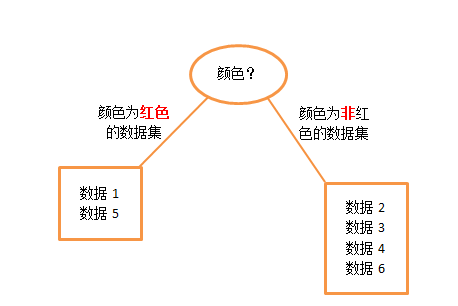1 数据集的基尼指数达到设定值，通俗的说，就是里面的类的纯度达到一定值。
2 数据集为空。

1 找一批已经分类正确的数据当做验证集，从最后一层节点开始，假设剪掉某个节点前后，用验证集数据去计算，剪掉前的准确率（对叶子进行计算）和剪掉后的准确率（对单节点进行计算），如果剪掉后的准确率没有下降，那么就减掉所有子节点，将当前父节点变成叶子。

2 采用损失评估。

C(T)是训练数据的预测误差，错误率，也可以用基尼指数来替代。
a是权衡训练数据的拟合度与模型的复杂度。
|T|是以T为根节点的所有叶子数量。

1 利用某个公式（基尼系数或信息增益）在所有属性里找出某个属性值，以此属性值作为划分点，能使其下每个子节点的数据集里的某个类占的比例尽量多，逐层建立树节点。

2 将建立好的决策树进行剪枝，如果剪掉某个节点后，准确率没有下降，那么就剪掉此节点。

Good good study, day day up!

Email

2023 mypcrun.com